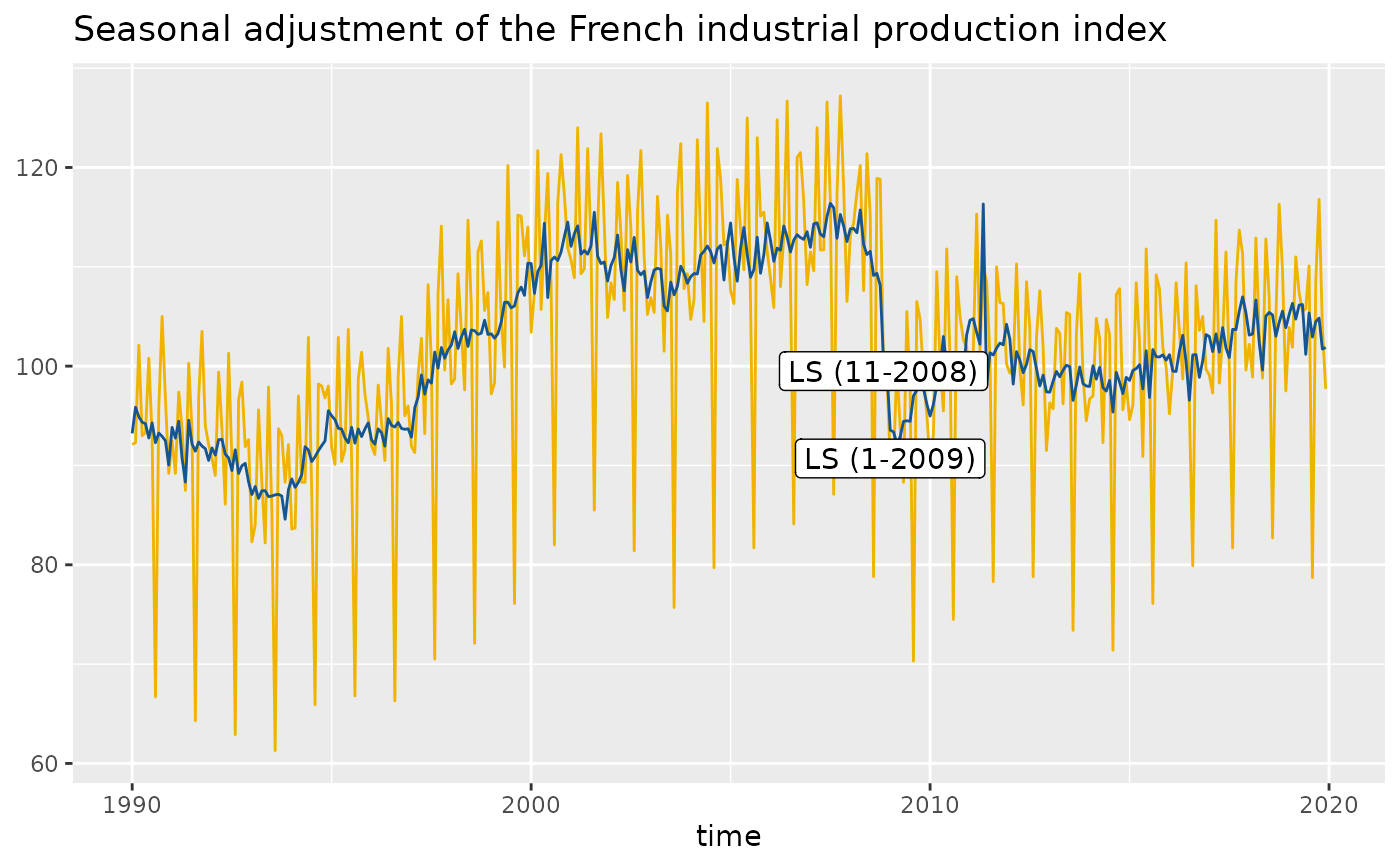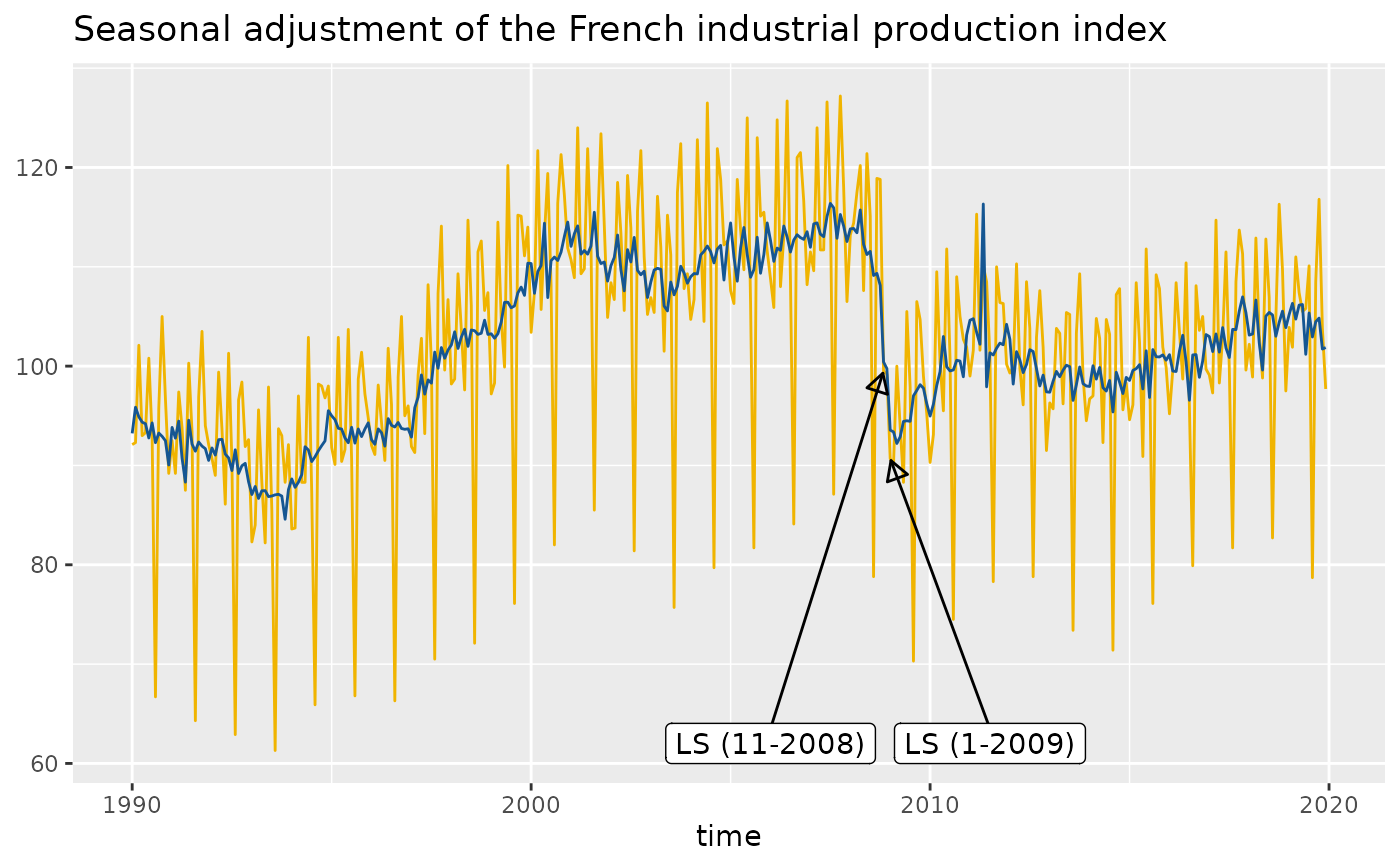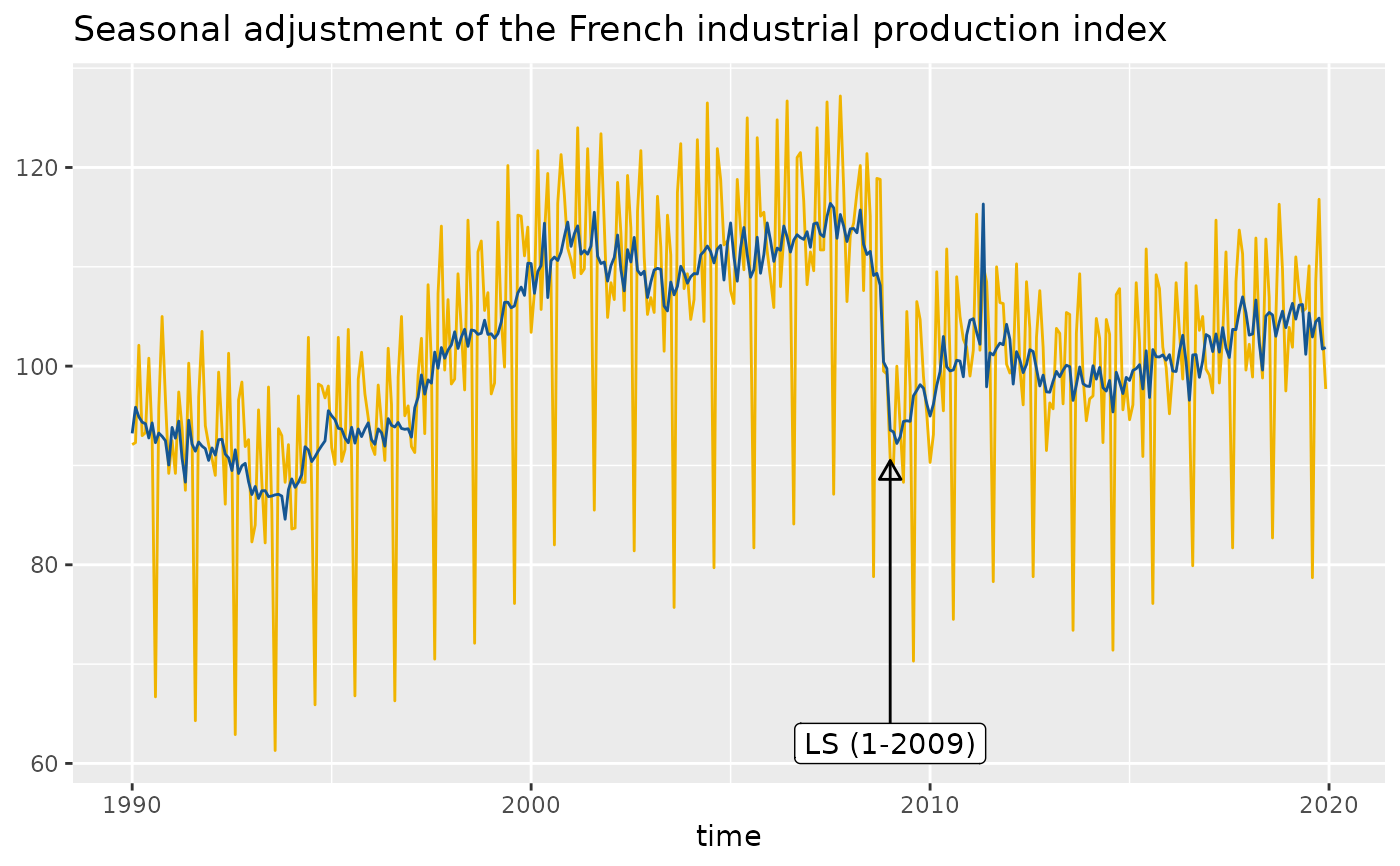Function to add directly to the plot the outliers used in the pre-adjustment process of the seasonal adjustment.

geom_outlier(
mapping = NULL,
data = NULL,
stat = "outlier",
geom = c("text", "label", "text_repel", "label_repel"),
position = "identity",
...,
method = c("x13", "tramoseats"),
spec = NULL,
frequency = NULL,
message = TRUE,
first_date = NULL,
last_date = NULL,
coefficients = FALSE,
digits = 1,
show.legend = NA,
inherit.aes = TRUE
)

## Arguments

mapping

Set of aesthetic mappings created by aes() or aes_(). If specified and inherit.aes = TRUE (the default), it is combined with the default mapping at the top level of the plot. You must supply mapping if there is no plot mapping.

data

A data.frame that contains the data used for the seasonal adjustment.

stat

The statistical transformation to use on the data for this layer, as a string.

geom

character. The geometric to use to display the data: GeomText (geom = "text", the default, see geom_text()); GeomLabel (geom = "label", see geom_label()); GeomTextRepel (geom = "text_repel", the default, see geom_text_repel()); GeomLabelRepel (geom = "label_repel", the default, see geom_label_repel()).

position

Position adjustment, either as a string, or the result of a call to a position adjustment function.

...

Other arguments passed on to layer(). They may be parameters of geom_text() (if geom = "text"), geom_label() (if geom = "label"), geom_text_repel() (if geom = "text_repel") or geom_label_repel() (if geom = "label_repel").

method

the method used for the seasonal adjustment. "x13" (by default) for the X-13ARIMA method and "tramoseats" for TRAMO-SEATS.

spec

the specification used for the seasonal adjustment. See x13() or tramoseats().

frequency

the frequency of the time series. By default (frequency = NULL), the frequency is computed automatically.

message

a boolean indicating if a message is printed with the frequency used.

first_date

A numeric specifying the first date from which the outliers are plotted. By default (first_date = NULL) the outliers are plotted from the beginning of the time series.

last_date

A numeric specifying the first date from which the outliers are plotted. By default (first_date = NULL) the outliers are plotted until the end of the time series.

coefficients

boolean indicating if the estimates coefficients are printed. By default coefficients = FALSE.

digits

integer indicating the number of decimal places to be used for numeric diagnostics. By default digits = 1.

show.legend

logical. Should this layer be included in the legends? NA, the default, includes if any aesthetics are mapped. FALSE never includes, and TRUE always includes. It can also be a named logical vector to finely select the aesthetics to display.

inherit.aes

If FALSE, overrides the default aesthetics, rather than combining with them.

## Details

With the parameter geom = "text", the outliers used in the pre-adjustment process of the seasonal adjustment are directly added to the plot. With geom = "label" a rectangle is drawn behind the names of the outliers, making them easier to read. The same with geom = "text_repel" or geom = "label_repel" but text labels are also repeled away from each other and away from the data points (see geom_label_repel()).

## Examples

p_sa_ipi_fr <- ggplot(data = ipi_c_eu_df, mapping = aes(x = date, y = FR)) +
geom_line(color =  "#F0B400") +
labs(title = "Seasonal adjustment of the French industrial production index",
x = "time", y = NULL)  +
geom_sa(color = "#155692", message = FALSE)

p_sa_ipi_fr + geom_outlier(geom = "label",
message = FALSE)# To have a more readable plot with outliers names that repeled away from each other
# and from the data points:
p_sa_ipi_fr +
geom_outlier(geom = "label_repel",
message = FALSE,
ylim = c(NA, 65),
arrow = arrow(length = unit(0.03, "npc"),
type = "closed", ends = "last"))# To only plot the outliers from a specific date (2009):
p_sa_ipi_fr +
geom_outlier(geom = "label_repel",
message = FALSE,
first_date = 2009,
ylim = c(NA, 65),
arrow = arrow(length = unit(0.03, "npc"),
type = "closed", ends = "last"))